Abstract: 数字图像处理：第33天
Keywords: 非锐化掩蔽(USM)

## 数学原理

1. 生成模板，$\bar{f}$ 表示 $f$ 的平滑结果：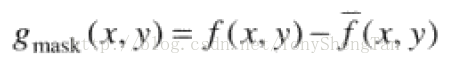2. 钝化模板按照一定比例与原图相加：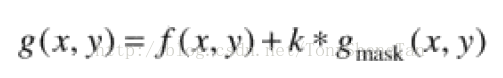其中 $k=1$ 时为非锐化掩蔽。
$k>1$时为高提升滤波。

## 示意图

观察示意图：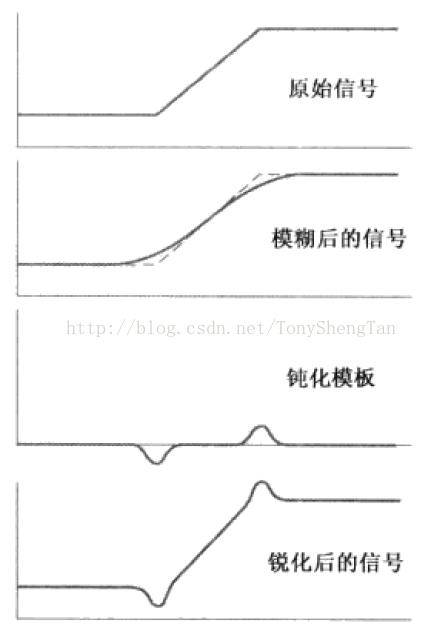算法基本步骤：

3. 平滑图像，边缘和突变被消减，而平滑部分不变（此处不适合采用边缘保持性好的平滑算法，代码中使用了均值滤波和高斯滤波）。

4. 原图像与平滑后图像相减，得到钝化模板。
5. 钝化模板的k倍与原图相加得到锐化结果。
值得注意的是，因为钝化模板有负值，所以，如果k选择过大的时候有可能图像产生负值## 结果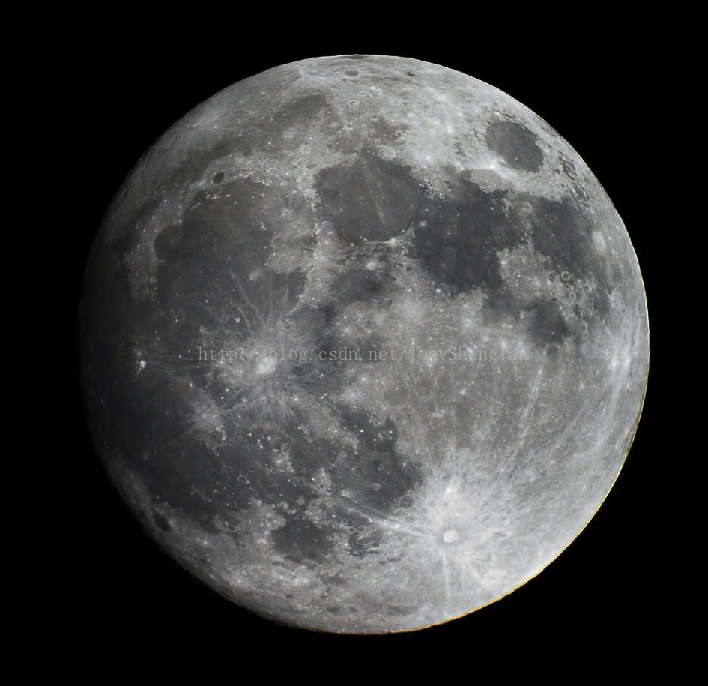$5\times 5$ ，$\sigma=1$ ，$k=1$ ：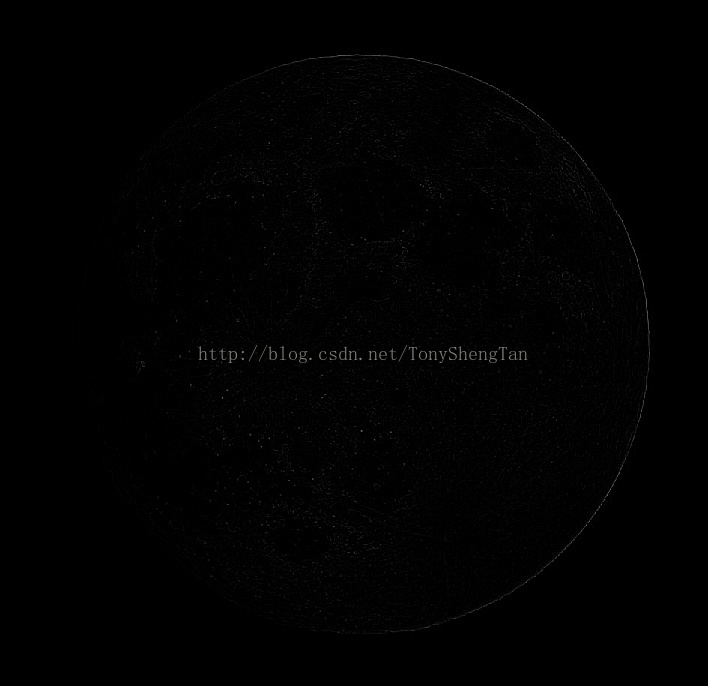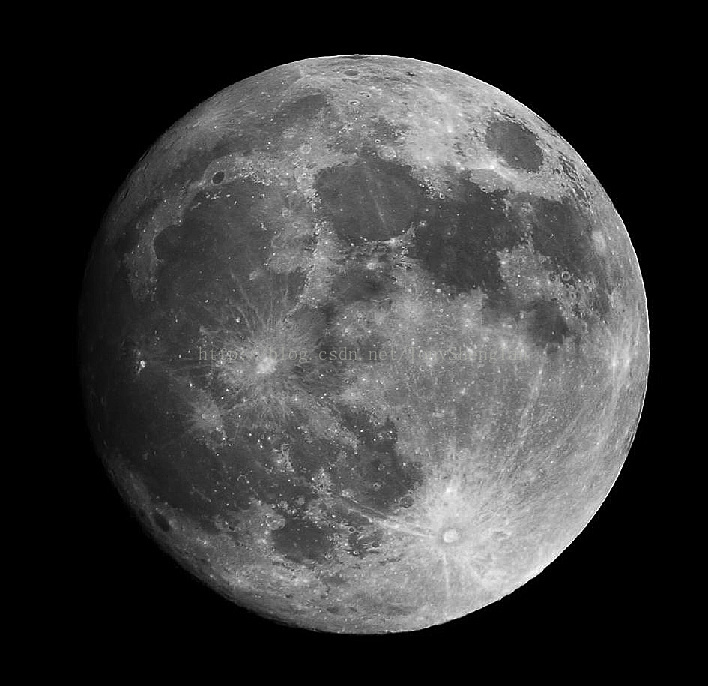$5\times 5$ ，$\sigma=1$ ，$k=2$：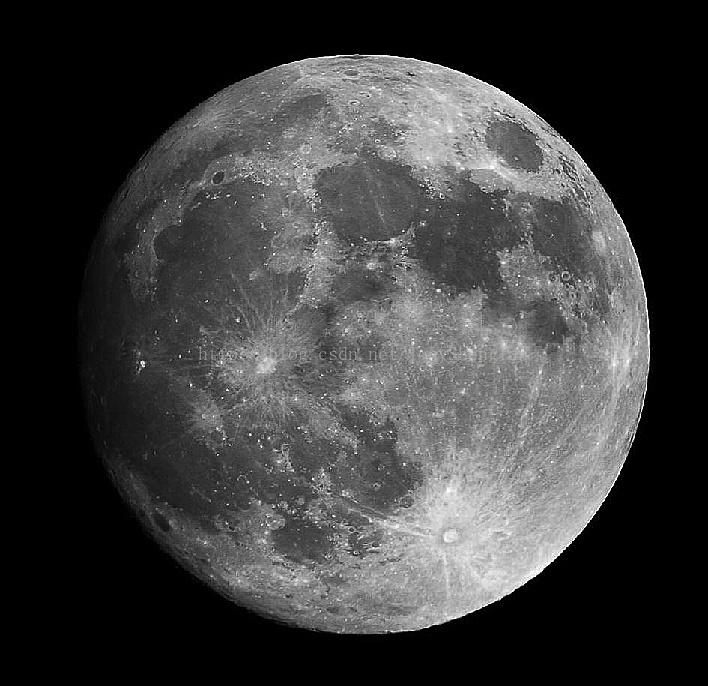$5\times 5$ ，$\sigma=2$ ，$k=1$：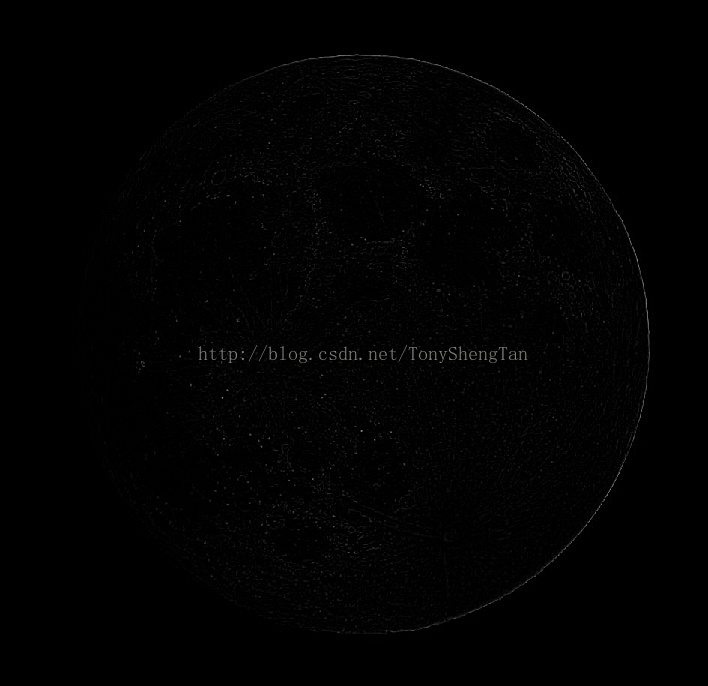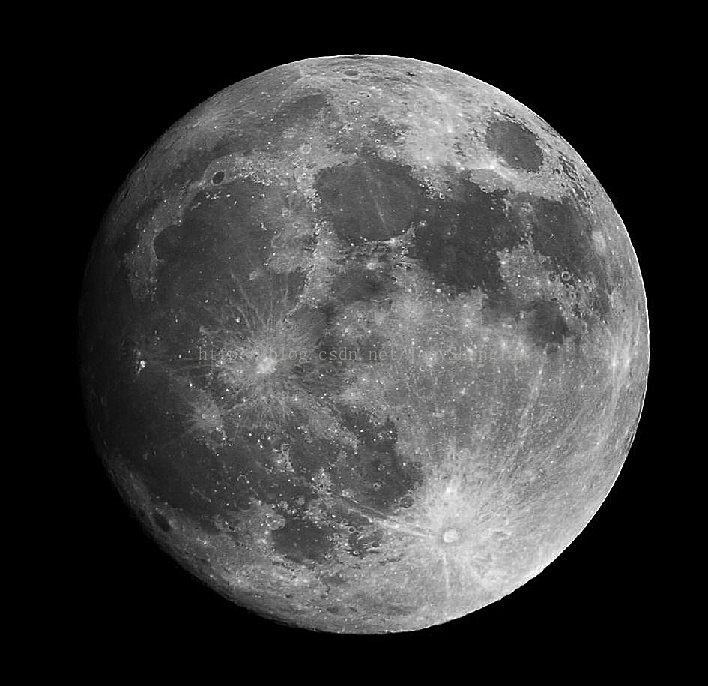$5\times 5$ ，$\sigma=2$ ，$k=2$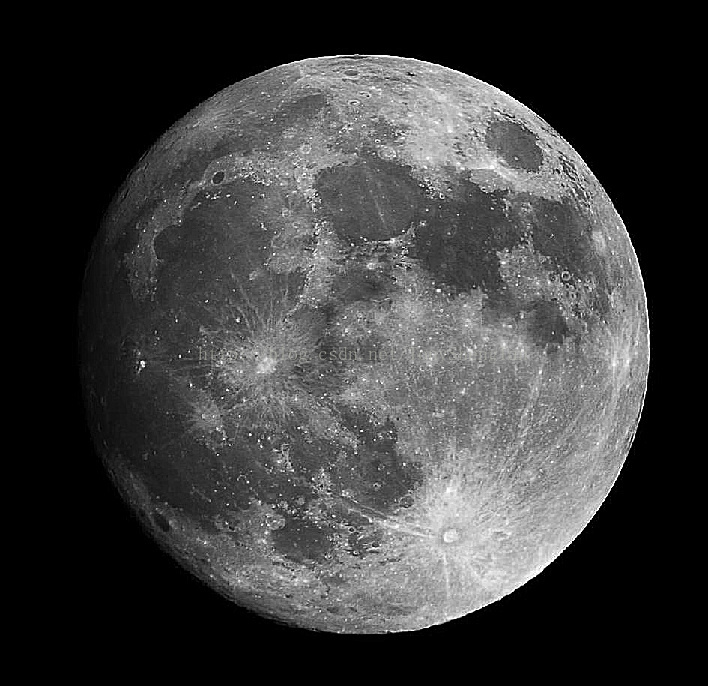$7\times 7$ ，$\sigma=1$ ，$k=1$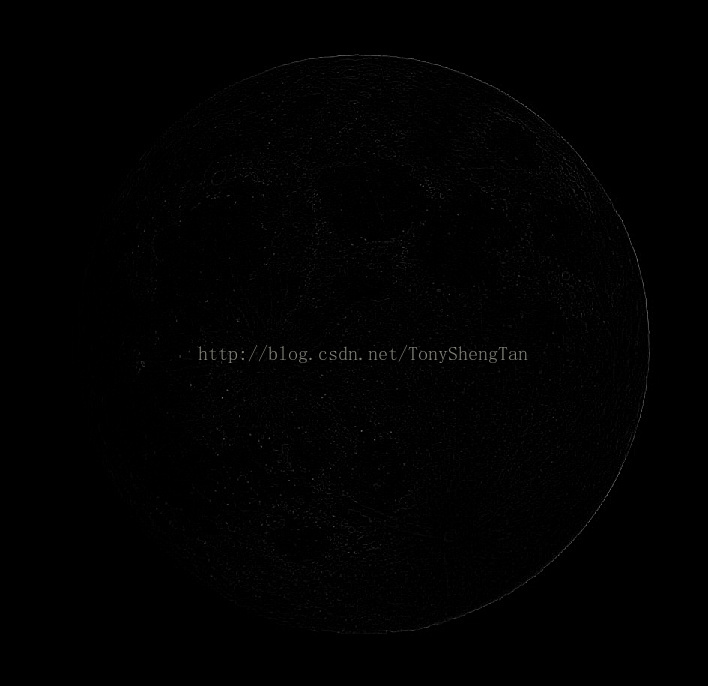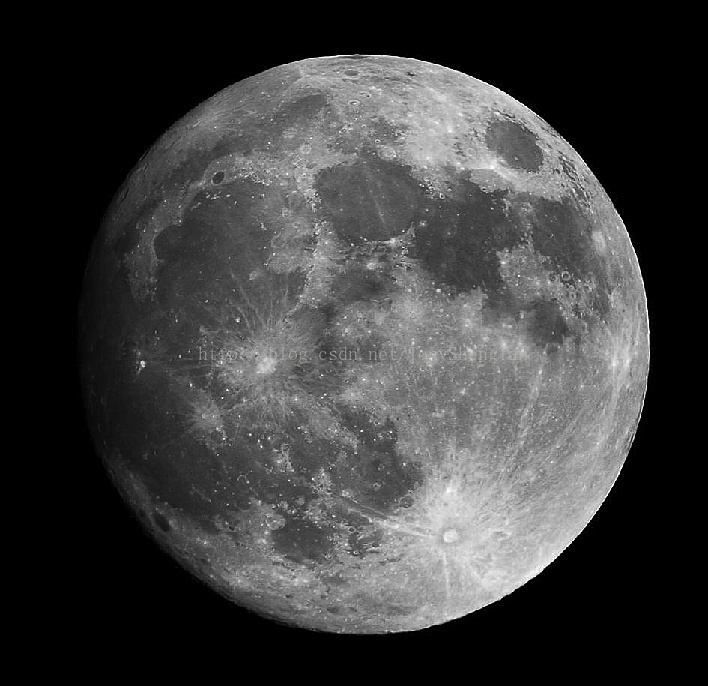$7\times 7$ ，$\sigma=1$ ，$k=2$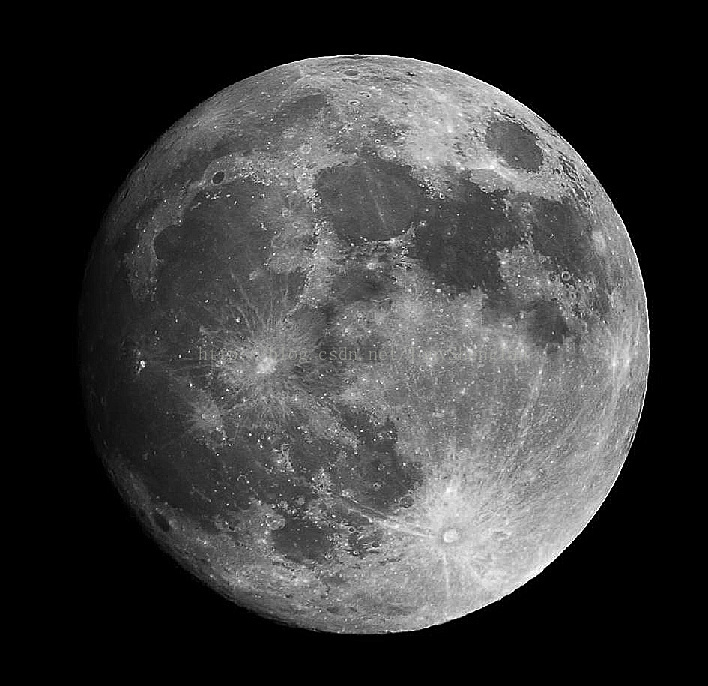$7\times 7$ ，$\sigma=2$ ，$k=1$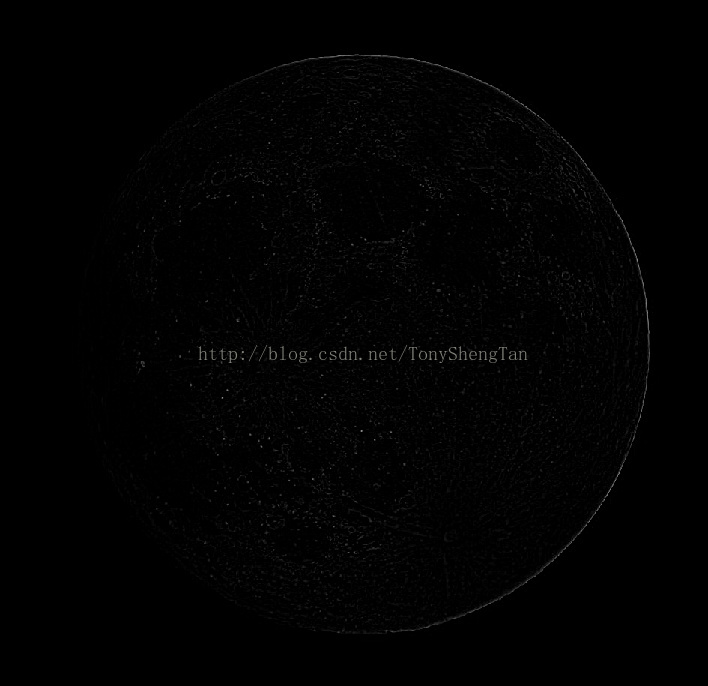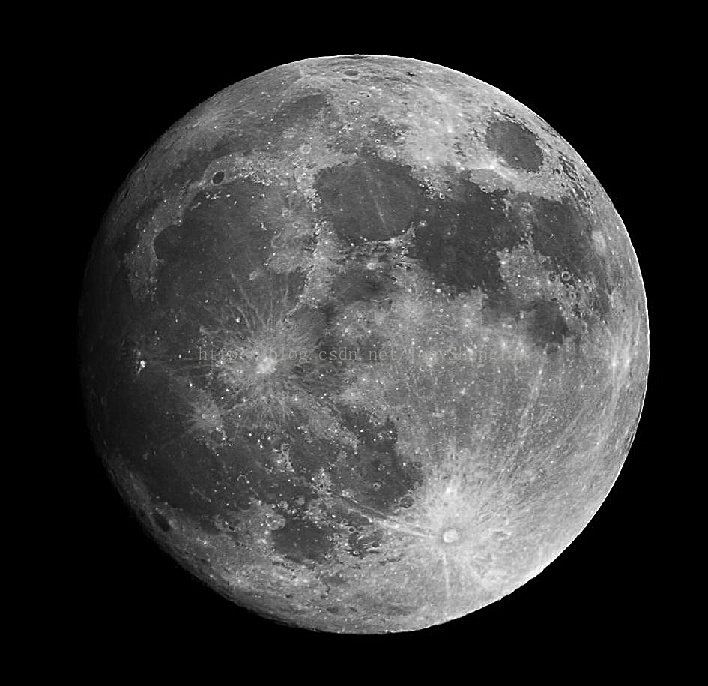$7\times 7$ ，$\sigma=2$ ，$k=2$：5x5，k=1：5x5，k=2：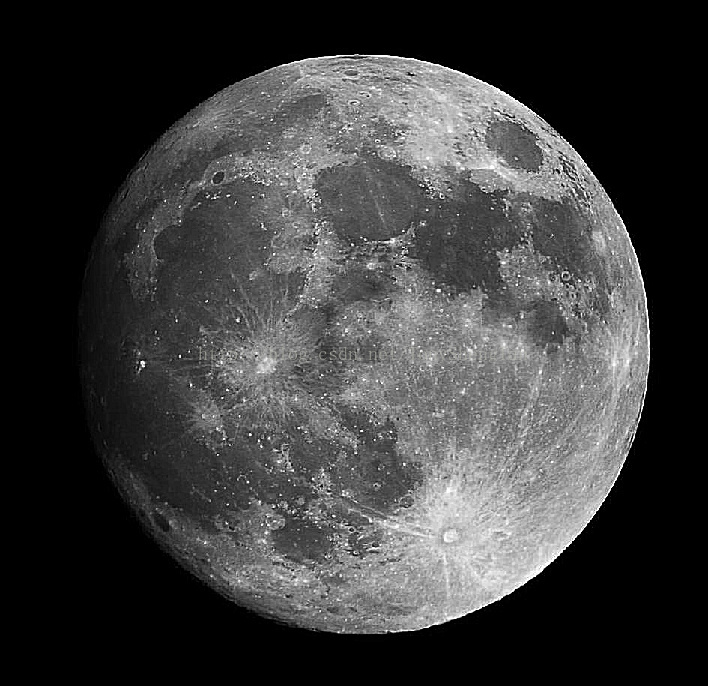7x7，k=1：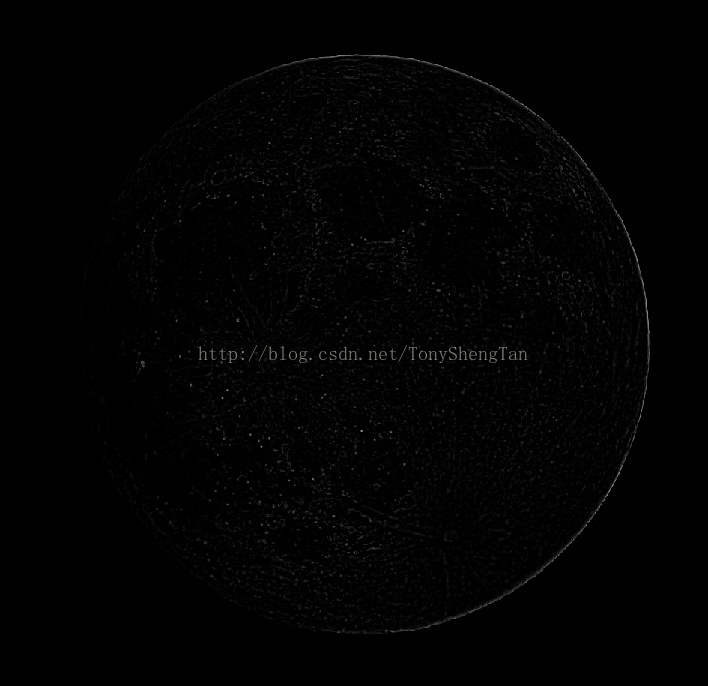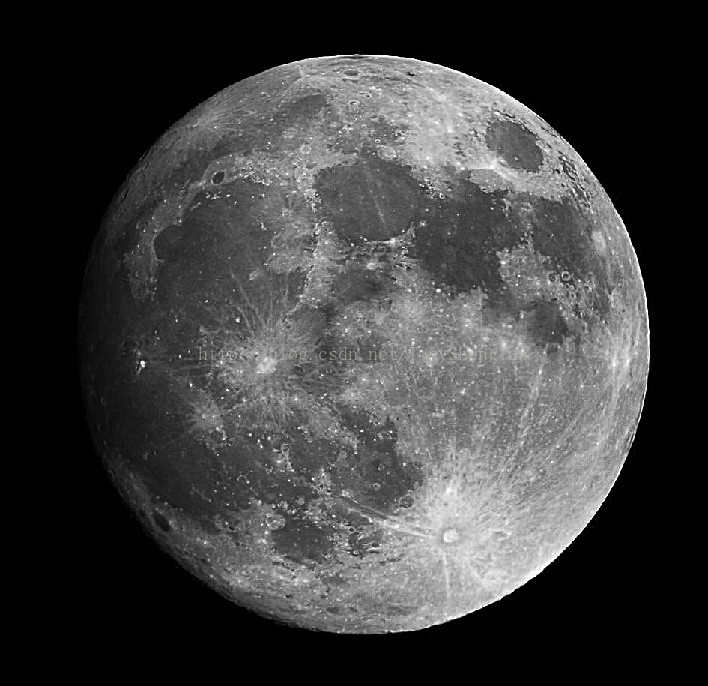7x7，k=2：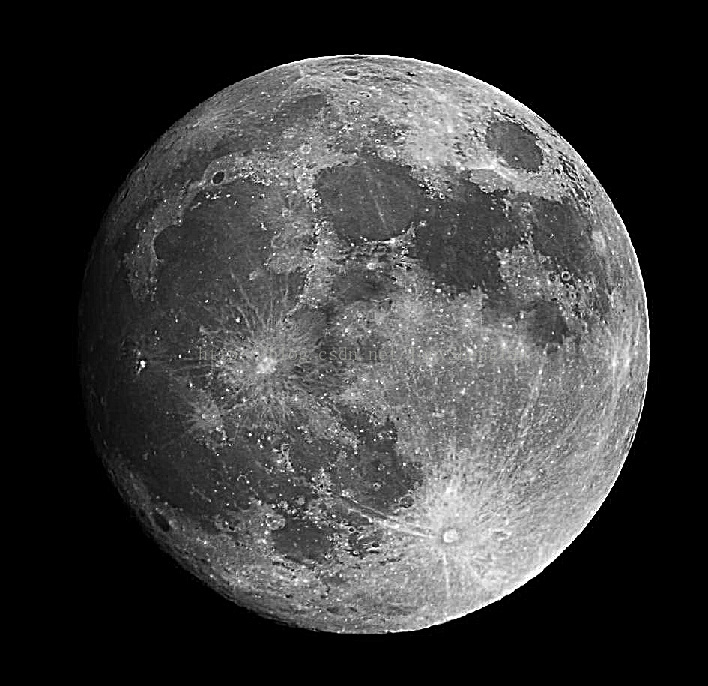## 总结

1. k越大对细节增强越明显。
2. 模板越大会导致增细节被增强的越宽，所以模板大小要适度。
3. 被平滑消除的边缘越多，锐化后增强的越多。
下一篇介绍下一阶微分算子
待续。。。

0%